### Thermodynamics Relations - Proof 12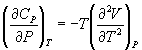By the proof given in Equn.4.4,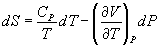→ (12.1)

This equation is similar to the form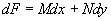→ (12.2)

where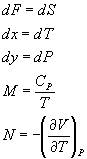For the differential equation 12.2, the criterion of exactness is given by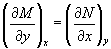i.e.,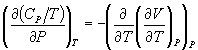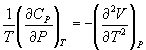→ (12.3)

Similarly starting from Equn.3.4,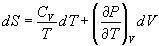it can be proved that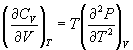→ (12.4)